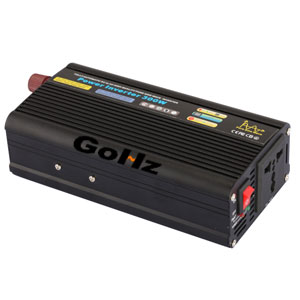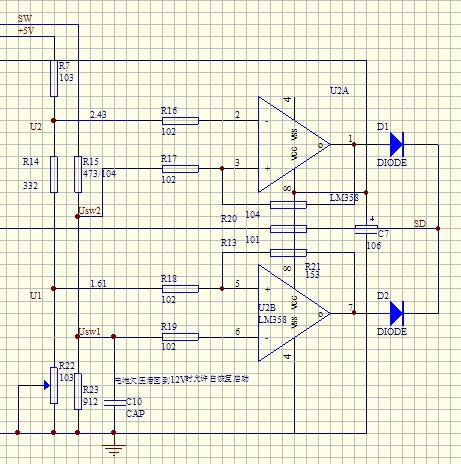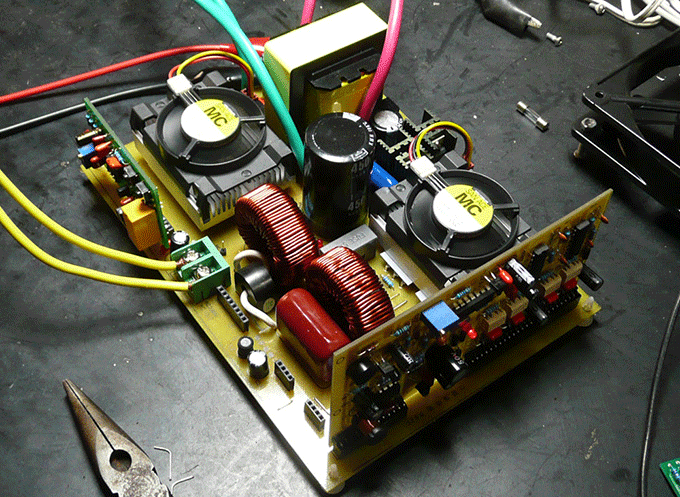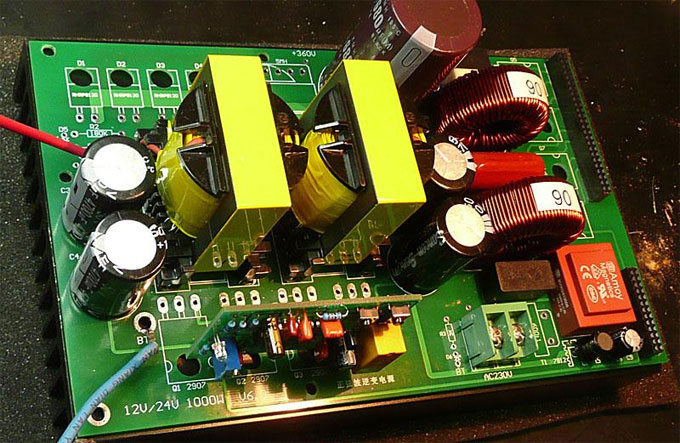Home » Basics » Power Inverter and Battery Life Calculation

# Power Inverter and Battery Life Calculation

There are a lot of letters and numbers printed on the battery, just find the words XXAH to know the battery capacity. Let me talk about the meaning of AH. A for ampere (amp.), is the current unit, H for hours (h). "Ah," the two letters together means "ampere per hour", referred to within an hour how many amps sustainable output. XX is usually in front of the two figures, namely the number of amps. For example, 45AH on behalf of this battery can be output12 volt 45 amps of current in an hour. As this battery can power output, we use 12 volts multiplied by 45 amps, draw 540 watts, which is the output power of the battery (theoretical value).
If the battery specification is 12V 50Ah, we multiplied 12V and 50A, obtained battery output power of 600 watts. If the efficiency of the inverter is 90%, then 90% then we multiplied by 600 watts, 540 watts draw. This means that your piece of the battery can push a maximum power output of 540W power inverter. Of course, you can also take "one step" type of procurement approach, which is to present its own regardless of the car with the battery specifications, and buy a power output of 800 watts power inverter. Then, in the first moment this allows the battery range, so in the future for a larger car and then uses the full power. Finally, the power inverter do not ask, for example, 100 watts is enough, then you can buy a small power inverter. Further, when determining the power of the inverter, there is an important principle that when using an inverter, not long-term full-load operation, which would significantly shorten the life of the inverter, the inverter failure rate while also significantly rise. We strongly recommend that users, it is best to use the inverter in not more than 85% of the rated power of the state.
Formula: Working hours=battery voltage×battery capacity×0.8×0.8÷appliance power
Example: 12V 60AH driven by a solenoid electrical 220V 100W
Working Hours=12V×60AH×0.8×0.9÷100=5.184h
NOTE: The battery discharge coefficient is 0.8, 0.9 is the conversion efficiency of the power inverter, the specific use of time and also about the performance of your old battery.Plz Calculate (7 - 4) =
(Your comment will show after approved.)

You may also like:Power Inverter Source

Featured Articles
How to Choose a Suitable Power ...How to select the inverter for an air conditioner, television, computer or the motor? How to match the battery? How long is the ...
12V 300W Uni-polar Isolation Pure Sine ...12v 300w uni-polar isolation of pure sine wave inverter Basic parameters Nominal power: 300W; continuous power: 250W; Peak power: ...
600w Pure Sine Wave Power Inverter ...I spent nearly a month design a 600w pure sine wave power inverter. The machine has the following characteristics: 1. SPWM drive ...
1000w 12V DC Home Power Inverter ...This power inverter is designed for 12v DC, but also can be connected to 24v DC, my goal is 800 watt, strive to 1000 watt pure ...